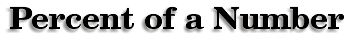If you sit in a shopping mall long enough, it is almost a certainty that you will hear someone say, "What is 25% of \$60 or what is 40% of \$30?"

 The first step in calculating the percent of numbers is to change the percent to a decimal. When you see the word "of" in word problems, that signals multiplication, so take the decimal and multiply by the number.Example: Find 25% of \$60. .25 x 60 =\$15 By changing the percent to a decimal and translating the word "of" to multiplication we have a simple solution. Example: Find 40% of \$30. .40 x 30 = \$12We can now discuss sales price and discount.   A suit is regularly priced at \$120, and it is marked 20% off the regular price. What is the new price for the suit? Follow the previous examples and begin by taking 20% of \$120. 20% of \$120 = .20 x 120 = \$24.00 We call \$24 the discount. We calculate the sales price by taking the regular price \$120 and subtracting the discount \$24… \$120 – \$24 = \$96 The sales price and cost of the dress is therefore \$96. This classic lawnmower costs \$85. What is its sale price? \$The new CD from Atomic Fish is called 33% Solution. If the solution was made up of 1800 ml, how many milliliters would constitute 33%?

Percent of Increase and Decrease
 writer:Kevin Gallagher

Percent